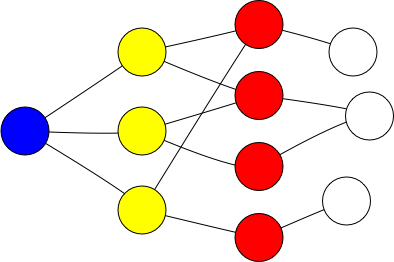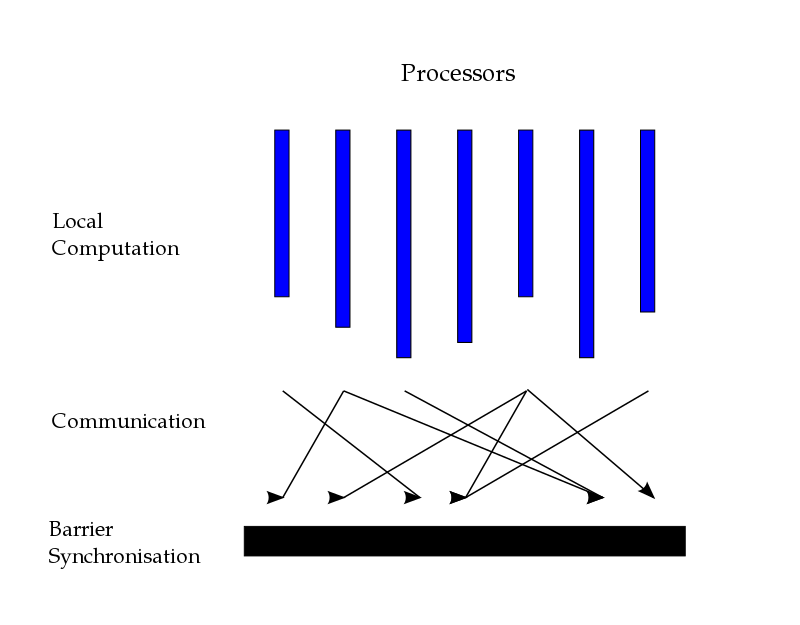# Processing Graphs

Graphs and other forms of hierarchical data structures appear every time a system models a dependency relationship. Common big graphs are:

• The social graph in social networking applications
• The web graph of linked pages
• The dependency graph in (software) package ecosystems

## Graph representations in short

A graph ($$G$$) comprises nodes ($$V$$) and edges ($$E$$). Both can carry metadata. We represent graphs as:

• Adjacency matrix: An $$n \times n$$ matrix $$M$$ ($$n = |V|$$) where a non-zero element $$M_{ij}$$ indicates and edge from $$V_i$$ to $$V_j$$
• Adjacency list: A List[(V, List[V])] where each tuple represents a node and its connections to other nodes.
• Edge list: A List[(V, V)] of node pairs that represents and edge

## Graph (sub-)structures

• Graph components: subgraphs in which any two vertices are connected to each other by paths.

• Strongly connected component: The largest sub-graph with a path from each node to every other node

• Triangles or polygons: sub-graphs with 3 or more nodes, where each node is connect to at most 2 others

• Spanning trees: A sub-graph that contains all nodes and the minimum number of edges

## Typical graph algorithms

• Traversal: Starting from a node, find all connected nodes
• Depth-first: Recursively follow all graph edges until all reachable nodes are visited
• Breadth-first: Follow graph edges per level; maintain a work-queue of visited nodes
• Node importance: Calculate the importance of a node relative to other nodes
• Centrality measures or PageRank
• Shortest paths
• Dijkstra’s algorithm or ‘traveling salesman’ approaches

## Typical graph applications

• Exploring the structure and evolution of communities or of systems of systems
• WWW
• Software libraries
• Recommending friends
• Recommending pages
• Community detection: sub-graphs with shared properties

## Approaches for graph processing

To process graphs, we can:

• Use SQL databases and recursive queries
• Use a graph database

For really big graphs, our options are somewhat limited

• Efficiently compress the graphs so that they fit in memory
• Use a message-passing architecture, like the bulk synchronous parallel model

# Typical graph applications

Not all applications need to process billions of nodes and trillions of edges. For small to medium sized graphs (< 500M edges), existing tools can go a long way.

## Graphs in SQL databases

CREATE TABLE nodes (
id INTEGER,
)

CREATE TABLE edges (
src INTEGER,
target INTEGER,
CONSTRAINT src_fkey
FOREIGN KEY (src) REFERENCES nodes(id),
CONSTRAINT target_fkey
FOREIGN KEY (target_id) REFERENCES nodes(id)
)

We model graphs as node pairs. Nodes and edges have metadata.

## SQL-based graph traversals

WITH RECURSIVE transitive_closure (a, b, distance, path) AS
(
SELECT a, b, 1 as distance, a || '->' || b AS path
FROM edges

UNION ALL

SELECT tc.a, e.b, tc.distance + 1, tc.path || '->' || e.b
FROM edges e
JOIN transitive_closure tc ON e.a = tc.b
WHERE a.metadata = ...   -- Traversal filters on nodes/edges
and tc.path NOT LIKE '%->' || e.b || '->%'
)
SELECT * FROM transitive_closure

Recursive queries have a starting clause that is called on and a recursion clause

## Example: Friend recommendationA simple social network

Given that we (blue node) are direct friends with the yellow nodes, we could recommend second level (red) friends as potential new connections.

## Recommending friends with SQL

WITH RECURSIVE transitive_closure (a, b, distance, path) AS
(
-- Find the yellow nodes
SELECT a, b, 1 as distance, a || '->' || b AS path
FROM edges
WHERE a = src -- the blue node

UNION ALL
-- Find the red nodes
SELECT tc.a, e.b, tc.distance + 1, tc.path || '->' || e.b
FROM edges e
JOIN transitive_closure tc ON e.a = tc.b
WHERE tc.path NOT LIKE '%->' || e.b || '->%'
AND tc.distance < 2 -- don't recurse into white nodes
)
SELECT a, b FROM transitive_closure
GROUP BY a, b
HAVING MIN(distance) = 2 -- only report red nodes

The base expression will find all directly connected nodes, while the second will recurse into their first level descendants.

## Graph databases

Graph databases are specialized RDBMs for storing recursive data structures and support CRUD operations on them, while maintaining transactional consistency (ACID or otherwise).

The most commonly used language for graph databases is Cypher, the base language for Neo4J.

MATCH (n:User{'foo'})
MATCH n-[:FRIENDS_WITH*2]-m
WHERE NOT n-[:FRIENDS_WITH]-m
RETURN m

# Big Graphs

Graphs are an inherently recursive data structures, which means that computations may have dependencies to previous computation steps (and thus they are not trivially parallelizable).

• Poor locality memory accesses
• Access patterns not very suitable for distribution
• Further complicated due to latency issues
• Little work to be done per node
• Applications mostly care about the edges

## Computation example: PageRank

Pagerank is a centrality measure based on the idea that nodes are important if multiple important nodes point to them. For node $$p_i$$, its Page rank is recursively defined as

$PR(p_i; 0) = \frac{1}{N} \\ PR(p_i; t+1) = \frac{1-d}{N} + d \sum_{p_j \in M(p_i)} \frac{PR (p_j; t)}{L(p_j)}$

where $$d$$ is a dumping factor (usually set 0.85) and $$M(p_i)$$ are the nodes pointing to $$p_i$$ We notice that each node updates other nodes by propagating its state.

## Simplified PageRank on Spark

val links: RDD[(V,List(E))] = ....cache()
var ranks = links.mapValues(v => 1.0)

for (i <- 1 to iters) {
}
ranks = contribs.reduceByKey(_ + _).mapValues(0.15 + 0.85 * _)
}

The computation is iterative and side-effecting and therefore non-parallelizable. To make it side-effect free, we need to write each step of the computation to external storage.

## Graph compression

Graph compression attempts to exploit graph characteristics to create an in-memory compressed edge representation.

Can compress billion edge graphs to ~3 bits / edge (down from 64).

Succinct representation enables good locality properties and fast traversals.

For more information look at this presentation by Paolo Boldi and Sebastiano Vigna.

## The bulk synchronous parallel model

The BSP model is a general model for parallel algorithms.

It assumes that a system has:

• multiple processors with fast local memory
• pair-wise communication between processors
• a barrier implementation (hardware or other) to synchronize super stepsThe BSP model

## BSP supersteps

BSP computation is organized in supersteps. A superstep comprises three phases:

• Local execution: Processors use own memory to perform computations on local data partitions
• Data exchange / remote communication: Exchange of data between processes
• Barrier synchronization: Processes wait until all processes finished communicating

## Pregel: Using BSP to process graphs

Pregel (by Google) is a distributed graph processing framework

• Pregel computations consist of a sequence of supersteps
• In a superstep, the framework invokes a user-defined function for each vertex
• Function specifies behaviour at a single vertex (V) and a single superstep (S)
• it can read messages sent to V in superstep (S-1)
• it can send messages to other vertices that will be read in superstep (S+1)
• it can modify the state of V and its outgoing edges

Open source implmentations: Apache Giraph and GraphX.

## Vertex centric approach

• Reminiscent of MapReduce
• User (i.e. algorithm developer) focus on a local action
• Each vertex is processed independently
• By design: well suited for a distributed implementation
• All communication is from superstep S to (S+1)
• No defined execution order within a superstep
• Free of deadlocks and data races

## Algorithm termination

BSP programs run until the programs stop themselves. Termination works as follows

• Superstep 0: all vertices are active
• All active vertices participate in the computation at each superstep
• A vertex deactivates itself by voting to halt
• No execution in subsequent supersteps
• A vertex can be reactivated by receiving a message

## Roles in a Pregel cluster

Graphs are stored as adjacency lists, partitioned (using hash partitioning) and distributed using a network filesystem

• Leader: Maintains a mapping between data partitions and cluster node. Implements the BSP barrier

• Worker: For each vertex, it maintains the following in memory:

• Current calculation value
• Queue of incoming messages
• State (active / inactive)

The worker applies all computationally intensive operations.

## A Pregel superstep

1. Workers combine incoming messages for all vertices.
• The combinator function updates the vertex state
2. If a termination condition has been met, the vertex votes to exclude itself for further iterations
3. (Optional) The vertex updates a global aggregator
4. Message passing:
• If receiving vertex is local: update its message queue
• Else wrap messages per receiving node and send them in bulk

## Pregel API in Spark

def pregel[A](
// Initialization message
initialMsg: A,
// Max super steps
maxIter: Int = Int.MaxValue,
activeDir: EdgeDirection = EdgeDirection.Out,
// Program to update the vertex
vprog: (VertexId, VD, A) => VD,
// Program to determine edges to send a message to
sendMsg: EdgeTriplet[VD, ED] => Iterator[(VertexId, A)],
// Program to combine incoming messages
mergeMsg: (A, A) => A
) : Graph[V, E]

Spark uses its underlying fault tolerance, check pointing, partitioning and communication mechanisms to store the graph. Halting is determined by examining if the vertex is sending / receiving messages.

## PageRank with Pregel/Spark

val pagerankGraph: Graph[Double, Double] = graph
.mapVertices((id, attr) => 1.0) // Initial Pagerank for nodes

def vertexProgram(id: VertexId, attr: Double, msgSum: Double): Double =
resetProb + (1.0 - resetProb) * msgSum
def sendMessage(id: VertexId, edge: EdgeTriplet[Double, Double]): Iterator[(VertexId, Double)] =
Iterator((edge.dstId, edge.srcAttr * edge.attr))
def messageCombiner(a: Double, b: Double): Double = a + b
val initialMessage = 0.0

// Execute Pregel for a fixed number of iterations.
Pregel(pagerankGraph, initialMessage, numIter)(
vertexProgram, sendMessage, messageCombiner)

From Pregel.scala in Apache Spark

## Fault tolerance

• The fault tolerance model is reminiscent of Spark.

• Periodically, the leader instructs the workers to save the state of their in-memory data to persistent storage

• Worker failure detected through keep-alive messages the leader issues to workers

• In case of failure, the leader reassigns graph partitions to live workers; they reload their partition state from the most recently available checkpoint